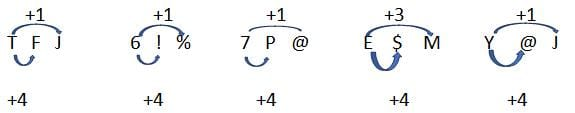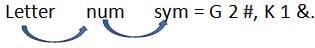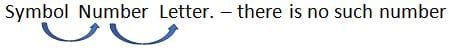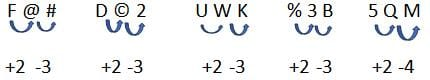CAT  >  Test: Letter Series Type - 5

# Test: Letter Series Type - 5

Test Description

## 15 Questions MCQ Test Logical Reasoning (LR) and Data Interpretation (DI) | Test: Letter Series Type - 5

Test: Letter Series Type - 5 for CAT 2022 is part of Logical Reasoning (LR) and Data Interpretation (DI) preparation. The Test: Letter Series Type - 5 questions and answers have been prepared according to the CAT exam syllabus.The Test: Letter Series Type - 5 MCQs are made for CAT 2022 Exam. Find important definitions, questions, notes, meanings, examples, exercises, MCQs and online tests for Test: Letter Series Type - 5 below.
Solutions of Test: Letter Series Type - 5 questions in English are available as part of our Logical Reasoning (LR) and Data Interpretation (DI) for CAT & Test: Letter Series Type - 5 solutions in Hindi for Logical Reasoning (LR) and Data Interpretation (DI) course. Download more important topics, notes, lectures and mock test series for CAT Exam by signing up for free. Attempt Test: Letter Series Type - 5 | 15 questions in 10 minutes | Mock test for CAT preparation | Free important questions MCQ to study Logical Reasoning (LR) and Data Interpretation (DI) for CAT Exam | Download free PDF with solutions
 1 Crore+ students have signed up on EduRev. Have you?
Test: Letter Series Type - 5 - Question 1

### Study the following arrangement and answer the questions below: 5 T J & A F 5 6 % Y J ! 7 @ M # P A 8 + E K A M \$ 4 5 I Which is the 3rd term to the left of the 15th term which is to the left of the 2nd term from the right end?

Detailed Solution for Test: Letter Series Type - 5 - Question 1

5 T J & A | F 5 6 % Y | J ! 7 @ M | # P A 8 + | E K A M \$ | 4 5 I
We divided series in group of each to save time.
Total elements – 28
2nd from right- 27 its 15 left = 27 – 15 = 12 its 3rd left = 12 – 3 = 9
Which is ‘%’.

Test: Letter Series Type - 5 - Question 2

### Study the following arrangement and answer the questions below: 5 T J & A F 5 6 % Y J ! 7 @ M # P A 8 + E K A M \$ 4 5 I How many such consonants are there each of which is followed by a vowel but is not preceded by a letter?

Detailed Solution for Test: Letter Series Type - 5 - Question 2

5 T J & A | F 5 6 % Y | J ! 7 @ M | # P A 8 + | E K A M \$ | 4 5 I
We divided series in group of each to save time.
Consonant followed by vowel but not preceded by letter-
#PA.

Test: Letter Series Type - 5 - Question 3

### Study the following arrangement and answer the questions below: 5 T J & A F 5 6 % Y J ! 7 @ M # P A 8 + E K A M \$ 4 5 I If all the symbols and vowels are dropped from the series, which will be 10th element from the right end?

Detailed Solution for Test: Letter Series Type - 5 - Question 3

5 T J & A | F 5 6 % Y | J ! 7 @ M | # P A 8 + | E K A M \$ | 4 5 I
We divided series in group of each to save time.
Series after given condition – 5 T J F 5 6 Y J 7 M P 8 K M 4 5
10th from right –Y.

Test: Letter Series Type - 5 - Question 4

Study the following arrangement and answer the questions below:
5 T J & A F 5 6 % Y J ! 7 @ M # P A 8 + E K A M \$ 4 5 I

If a vowel gets 1 point, a symbol gets 2 points, all letters that are not vowel get 3 points and the number gets the same number of points that they represent then what is the sum of the series?

Detailed Solution for Test: Letter Series Type - 5 - Question 4

5 T J & A | F 5 6 % Y | J ! 7 @ M | # P A 8 + | E K A M \$ | 4 5 I
We divided series in group of each to save time.
Sum – 86.

Test: Letter Series Type - 5 - Question 5

Study the following arrangement and answer the questions below:
5 T J & A F 5 6 % Y J ! 7 @ M # P A 8 + E K A M \$ 4 5 I

Four of the five sequence are alike in some manner. Find the odd one out?

Detailed Solution for Test: Letter Series Type - 5 - Question 5

5 T J & A | F 5 6 % Y | J ! 7 @ M | # P A 8 + | E K A M \$ | 4 5 I
We divided series in group of each to save time.Test: Letter Series Type - 5 - Question 6

Study the following arrangement and answer the questions below:
2 R 8 C F 8 E \$ G 2 # 4 9 L C % B < K 1 & A W ? P E = Q @ 7 F 6

If the above sequence is written in reverse order, then which element will be 5th to the right of the 15th element from the right end?

Detailed Solution for Test: Letter Series Type - 5 - Question 6

2 R 8 C F 8 E \$ | G 2 # 4 9 | L C % B < | K 1 & A W | ? P E = Q | @ 7 F 6
15th from right – 32 + 1 – 15 = 18th from left. Its 5th right = 18 + 5 = 23rd if series reversed – 1 + 32 -23 = 10th. Which is 2.

Test: Letter Series Type - 5 - Question 7

Study the following arrangement and answer the questions below:
2 R 8 C F 8 E \$ G 2 # 4 9 L C % B < K 1 & A W ? P E = Q @ 7 F 6

How many such numbers are there in the above sequence, each of which is immediately preceded by a constant and immediately followed by a symbol?

Detailed Solution for Test: Letter Series Type - 5 - Question 7

2 R 8 C F 8 E \$ | G 2 # 4 9 | L C % B < | K 1 & A W | ? P E = Q | @ 7 F 6Test: Letter Series Type - 5 - Question 8

Study the following arrangement and answer the questions below:
2 R 8 C F 8 E \$ G 2 # 4 9 L C % B < K 1 & A W ? P E = Q @ 7 F 6

Which element is 7th to the left of 16th element from the left in the above sequence?

Detailed Solution for Test: Letter Series Type - 5 - Question 8

2 R 8 C F 8 E \$ | G 2 # 4 9 | L C % B < | K 1 & A W | ? P E = Q | @ 7 F 6
7th left to 16th from left – 16 – 7 = 9th which is G.

Test: Letter Series Type - 5 - Question 9

Study the following arrangement and answer the questions below:
2 R 8 C F 8 E \$ G 2 # 4 9 L C % B < K 1 & A W ? P E = Q @ 7 F 6

If first half of the series is written in reverse order, then which element will be 21st from the right end?

Detailed Solution for Test: Letter Series Type - 5 - Question 9

2 R 8 C F 8 E \$ | G 2 # 4 9 | L C % B < | K 1 & A W | ? P E = Q | @ 7 F 6
21st from right means – second half + 5th from right in first half, that means 5th from left in original series which if – F

Test: Letter Series Type - 5 - Question 10

Study the following arrangement and answer the questions below:
2 R 8 C F 8 E \$ G 2 # 4 9 L C % B < K 1 & A W ? P E = Q @ 7 F 6

If the second half of the series is written in reverse order, then which element will be 21st from the right end?

Detailed Solution for Test: Letter Series Type - 5 - Question 10

2 R 8 C F 8 E \$ | G 2 # 4 9 | L C % B < | K 1 & A W | ? P E = Q | @ 7 F 6
21st from right means- second half +5th from right in first half,
That means 12th from left which is 4.

Test: Letter Series Type - 5 - Question 11

Study the following arrangement and answer the questions below:
E 4 B % R 3 A 6 # F H @ I 2 D 9 © K U \$ W 1 M P 5 * Q 8 T

If all the numbers are dropped from the above arrangement, Which one of the following will be the 9th to the left of W?

Detailed Solution for Test: Letter Series Type - 5 - Question 11

E 4 B % R 3 A 6 | # F H @ I | 2 D 9 © K | U \$ W 1 M | P 5 * Q 8 T |
W = 21st , its 9th left = 21 - 9 = 12, numbers in between = 2,
Therefore element is F.

Test: Letter Series Type - 5 - Question 12

Study the following arrangement and answer the questions below:
E 4 B % R 3 A 6 # F H @ I 2 D 9 © K U \$ W 1 M P 5 * Q 8 T

How many such numbers are there in the above arrangement each of which is immediately preceded by a symbol and immediately followed by a letter?

Detailed Solution for Test: Letter Series Type - 5 - Question 12

E 4 B % R 3 A 6 | # F H @ I | 2 D 9 © K | U \$ W 1 M | P 5 * Q 8 T |Test: Letter Series Type - 5 - Question 13

Study the following arrangement and answer the questions below:
E 4 B % R 3 A 6 # F H @ I 2 D 9 © K U \$ W 1 M P 5 * Q 8 T

Which of the following is 5th to the right of 18th from the right?

Detailed Solution for Test: Letter Series Type - 5 - Question 13

E 4 B % R 3 A 6 | # F H @ I | 2 D 9 © K | U \$ W 1 M | P 5 * Q 8 T |
18th from right = 29 + 1 – 18 = 12th from left, its 5th right = 12 + 5 = 17th = ©.

Test: Letter Series Type - 5 - Question 14

Study the following arrangement and answer the questions below:
E 4 B % R 3 A 6 # F H @ I 2 D 9 © K U \$ W 1 M P 5 * Q 8 T

Which of the following is 4th to the right of 12th from the left end?

Detailed Solution for Test: Letter Series Type - 5 - Question 14

4 B % R 3 A 6 | # F H @ I | 2 D 9 © K | U \$ W 1 M | P 5 * Q 8 T |
4th right to 12th from left = 12 + 4 = 16th = 9.

Test: Letter Series Type - 5 - Question 15

Study the following arrangement and answer the questions below:
E 4 B % R 3 A 6 # F H @ I 2 D 9 © K U \$ W 1 M P 5 * Q 8 T

Four of the below five are alike in a certain way. Find the odd one?

Detailed Solution for Test: Letter Series Type - 5 - Question 15

4 B % R 3 A 6 | # F H @ I | 2 D 9 © K | U \$ W 1 M | P 5 * Q 8 T |## Logical Reasoning (LR) and Data Interpretation (DI)

96 videos|87 docs|119 tests
 Use Code STAYHOME200 and get INR 200 additional OFF Use Coupon Code
Information about Test: Letter Series Type - 5 Page
In this test you can find the Exam questions for Test: Letter Series Type - 5 solved & explained in the simplest way possible. Besides giving Questions and answers for Test: Letter Series Type - 5, EduRev gives you an ample number of Online tests for practice

## Logical Reasoning (LR) and Data Interpretation (DI)

96 videos|87 docs|119 tests

### How to Prepare for CAT

Read our guide to prepare for CAT which is created by Toppers & the best Teachers